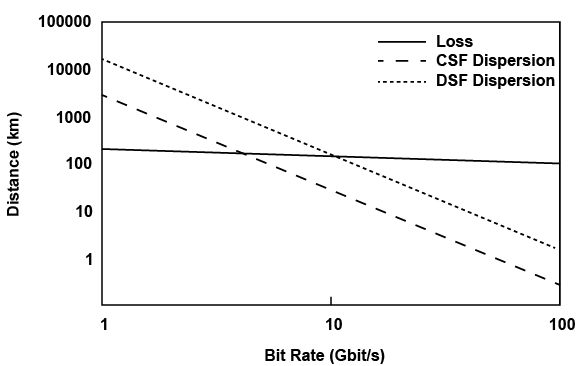# Optical Fiber Maximum Transmission Distance Limited by Attenuation and Dispersion (Without Amplifier)

In this tutorial, we will discuss the maximum distance that a fiber cable can transmit without an amplifier or repeater. This distance is limited by the fiber’s attenuation (loss) and dispersion (pulse widening). In practice, fiber link distance can be extended by using fiber amplifiers and dispersion compensators.

>> Fiber Distance Limit by Attenuation

For a practical fiber optic communication link, the Bit Error Ratio (BER) must be less than 10-9. Based on this BER requirement, there must be a minimum number of photons per bit of information. Let’s assume this minimum number is Np, then for a bit rate B, the minimum average optical power received Pr is: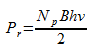where hv is the energy of each received photon.

If α represents the loss coefficient of the fiber in dB/km, then for an input power of Pi, the optical power at any length L of the fiber is given by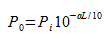So, if the received power is at least Pr, then the maximum permissible fiber length would be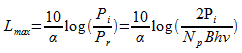Note that the maximum loss-limited fiber length decreases with increased data rate B.

OK. Let’s look at an example to further clarify this concept.

Consider a fiber link operating at 1300nm with a fiber loss of α = 0.4 dB/km. The input optical power is 1 mW and the minimum number of photons per bit of information Np is 1000. If the data rate is 2.5 Gbit/s, what is the maximum fiber length limited by fiber attenuation?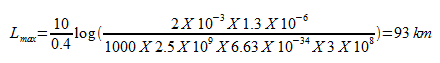>> Fiber Distance Limit by Fiber Dispersion

Optical pulse widening due to fiber dispersion is the other important limit on optical fiber’s maximum span distance without an amplifier.

The result of pulse widening is overlap between adjacent pulses, and this overlap causes increased detection error on the receiver side.

A popularly used criterion for the maximum allowed pulse dispersion is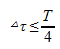where T is the digital bit time duration.  We can change the above equation to expression of data bit rate B, where B = 1/T.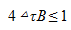For a single mode fiber, the dispersion is given by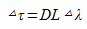where D is the dispersion coefficient, L is the fiber length, and △λ is the spectral width of the light source.

Multilongitudinal Laser

In the case of modulation of multilongitudinal mode laser (FP laser), the spectral width of the source is much larger than the spectrum due to modulation. So we get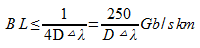For a conventional single mode fiber with zero dispersion at 1300nm, we take as typical parameters

△λ = 1nm, D = 1 ps/km nm

We get

BL ≤ 250 Gb/s km

This means that at 2.5 Gb/s, the maximum distance is 100 km with no amplifier.

However, if we operate this fiber at 1550nm, where D is about 16 ps/km nm, still assume △λ = 1nm, we get

BL ≤ 15 Gb/s km

This means at 2.5 Gb/s, the maximum distance is only 6 km.

Single Frequency Laser (DFB Laser)

For a single frequency single mode laser, the spectral width is also much larger than the inherent source spectral width due to digital modulation.

Now if τ0 is the temporal full width of the input pulse, then the spectral width is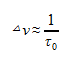which in terms of wavelength becomes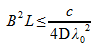Note that in this case, doubling the data rate B would reduce the maximum length L by a factor of 4.

For a conventional single mode fiber with zero dispersion at 1300nm, with D about 1 ps/km nm, we get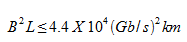So, at 2.5 Gbit/s, L ≤ 7040 km, at 10 Gbit/s, L ≤ 440km.

Again if we operate this conventional single mode fiber at 1550n, with D about 16 ps/km nm, we get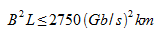So, at B = 2.5 Gbit/s, we have L ≤ 440 km, and at B = 10 Gbit/s, we have L ≤ 27.5 km. We can see the limitation set by fiber dispersion.

The following graph shows the maximum fiber length limited by pulse dispersion as well as fiber attenuation in single mode fiber.